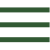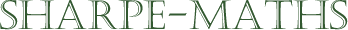Previous topic: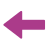Current topic:
Introduction to fractions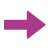Next topic:
Equivalent fractions & cancelling

### Fractions

Fractions are hard. There is no way of getting around this. They are difficult. Now once you've got them cracked, they will seem easy enough but until that point, they are hard. So, to quote the late lamented Douglas Adams, "Don't Panic!".

In the image to the right, you see a green circle divided into ${1 \over 2}$s (halves).
Next to it, you see a blue circle divided into ${1 \over 4}$s (quarters)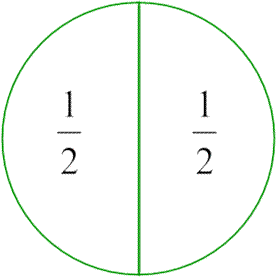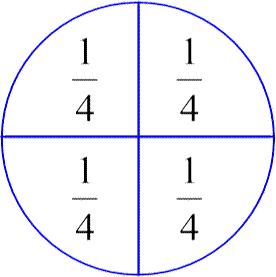You should notice that the halves are a completely different shape and size from each of the quarters. In fact, if you look carefully, you will see that the halves are exactly twice the size of the quarters. This means that $${1 \over 4} \times 2 = {2 \over 4} = {1 \over 2}.$$ This shows that doubling a quarter will give us a half, and we can also say that $${1 \over 4} + {1 \over 4} = {2 \over 4} = {1 \over 2}.$$ which is the same statement as before but with the multiplication reduced to its adding equivalent.

It is important to note that while the notation with fractions is a bit weird, at least the basic ideas of arithmetic, such as that multiplication is repeated adding, still holds true.

We can now say that the denominator tells us about the shape of the circle part. So a half is a semi-circle and a quarter is a quarter-circle. Below there are some other ways of dividing up a circle. You should notice that the shapes of each one is slightly different. It is not a coincidence that maths teachers frequently use pizzas as a way of getting students attention when covering this stuff.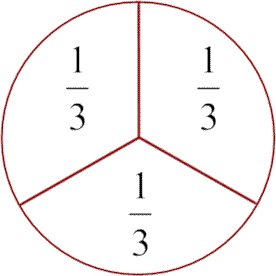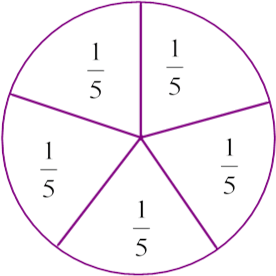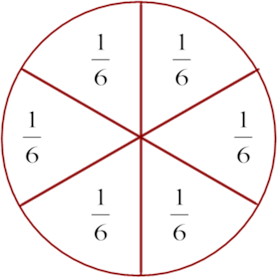We can also say that the numerators tell us about how many of these variously shaped parts, we have. So if you take $3$ of these $1 \over 5$ things, we have $3 \over 5$. Likewise if we take $3$ of the $1 \over 6$ things, we end up with $3 \over 6$ which, if you look at the shape that makes, gives us $1 \over 2$. This means that ${1 \over 2} = {2 \over 4} = {3 \over 6}$. These are called equivalent fractions. Fractions which look different, but ultimately mean exactly the same thing.

In the next section, we will be looking in more depth at Equivalent fractions.